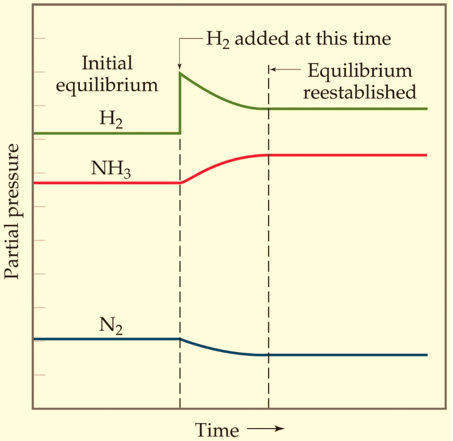# Le Chatelier’s principle

Equilibrium:Le Chatelier’s principle

Le Chatelier’s principle describes how a system atequilibrium reacts to changes (stresses) in system conditions. It states that if a system at equilibrium is disturbed by a change in temperature, pressure, or one of the components concentration, the system will transfer its equilibrium place to counteract the outcome of the disturbance. In other words, when stress is imposedon a system the system will respond to counteract the stress.

When a system is perturbed by changing the concentration, pressure, temperature or adding chemicals, the system works in a way that equilibrium is reestablished

Reaction accommodates the change in products, reactants, temperature, pressure, etc. and the rates of forward and reverses reactions re-equilibrate. Note that, presence of a catalyst does not shift the equilibrium, it merely lowers the activation energy to increase reaction rate which can be in any direction.

If the concentration of a reactant is increased, the system will react to decrease the amount of that reactant (shifts right; forward reaction occurs). If the concentration of a reactant is decreased, the system will react to make more of that reactant (shifts left; reverse reaction occurs). If the concentration of a product is increased, the system will react to decrease the amount of that product (shifts left; reverse reaction occurs).

If the pressure is increased, the system will react to decrease the pressure. If the pressure is decreased, the system will react to increase the pressure.

If the temperature is increased, the system will react to decrease the temperature by favouring the endothermic reaction. If the temperature is decreased, the system will react to increase the temperature by favouring the exothermic reaction.

The transformation of nitrogen and hydrogen into ammonia (NH3) is of tremendous significance in agriculture, where ammonia-based fertilizers are of utmost importance. It is a reversible reaction and the production of ammonia is based on analogies of Le Chatelier’s principle. This process is called the Haber process.

Consider the following reaction:

N2, H2, and NH3 are at equilibrium in a container at 500°C.Figure 1: Haber Process

In a case where more nitrogen is added to the reactor, the equilibrium shifts to the right to produce more ammonia. In a case where the reactor is compressed, the equilibrium shifts to right since 4 moles of gases are present in the left side and 2 moles of gas are present in the right side. So the reaction shifts to the right to balance the pressure difference. Where the temperature increase, the equilibrium shifts to left since the forward reaction is exothermic while the backward reaction is endothermic. Hence, the backward reaction becomes more favourable. Alternatively, when an inert gas such as Helium is added to the reactor at constant volume, no change to the equilibrium happens. Helium is not a reactant or product. Adding helium (at constant V) does not change PN2, PH2 or PNH3. Hence the equilibrium will not shift. But when helium is added at constant total pressure, the equilibrium shifts to left because PN2 + PH2 + PNH3 must decrease and there are more overall gas content and pressure in the reactor.